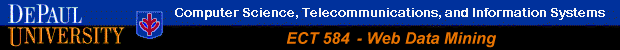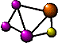Classification via Decision Trees in WEKA

 The following guide is based WEKA version 3.4.1 Additional resources on WEKA, including sample data sets can be found from the official WEKA Web site.

This example illustrates the use of C4.5 (J48) classifier in WEKA. The sample data set used for this example, unless otherwise indicated, is the bank data available in comma-separated format (bank-data.csv). This document assumes that appropriate data preprocessing has been perfromed. In this case ID field has been removed. Since C4.5 algorithm can handle numeric attributes, there is no need to discretize any of the attributes. For the purposes of this example, however, the "Children" attribute has been converted into a categorical attribute with values "YES" or "NO".

WEKA has implementations of numerous classification and prediction algorithms. The basic ideas behind using all of these are similar. In this example we will use the modified version of the bank data to classify new instances using the C4.5 algorithm (note that the C4.5 is implemented in WEKA by the classifier class: `weka.classifiers.trees.J48`). The modified (and smaller) version of the bank data can be found in the file "bank.arff" and the new unclassified instances are in the file "bank-new.arff".

As usual, we begin by loading the data into WEKA, as seen in Figure 20:

Figure 20

Next, we select the "Classify" tab and click the "Choose" button to select the J48 classifier, as depicted in Figures 21-a and 21-b. Note that J48 (implementation of C4.5 algorithm) does not require discretization of numeric attributes, in contrast to the ID3 algorithm from which C4.5 has evolved.

Figure 21-a     Figure 21-b

Now, we can specify the various parameters. These can be specified by clicking in the text box to the right of the "Choose" button, as depicted in Figure 22. In this example we accept the default values. The default version does perform some pruning (using the subtree raising approach), but does not perform error pruning. The selected parameters are depicted in Figure 22.

Figure 22

Under the "Test options" in the main panel we select 10-fold cross-validation as our evaluation approach. Since we do not have separate evaluation data set, this is necessary to get a reasonable idea of accuracy of the generated model. We now click "Start" to generate the model. The ASCII version of the tree as well as evaluation statistics will appear in the eight panel when the model construction is completed (see Figure 23).

Figure 23

We can view this information in a separate window by right clicking the last result set (inside the "Result list" panel on the left) and selecting "View in separate window" from the pop-up menu. These steps and the resulting window containing the classification results are depicted in Figures 24-a and 24-b.

Figure 24-a     Figure 24-b

Note that the classification accuracy of our model is only about 69%. This may indicate that we may need to do more work (either in preprocessing or in selecting the correct parameters for classification), before building another model. In this example, however, we will continue with this model despite its inaccuracy.

WEKA also let's us view a graphical rendition of the classification tree. This can be done by right clicking the last result set (as before) and selecting "Visualize tree" from the pop-up menu. The tree for this example is depicted in Figure 25. Note that by resizing the window and selecting various menu items from inside the tree view (using the right mouse button), we can adjust the tree view to make it more readable.

Figure 25

We will now use our model to classify the new instances. A portion of the new instances ARFF file is depicted in Figure 26. Note that the attribute section is identical to the training data (bank data we used for building our model). However, in the data section, the value of the "pep" attribute is "?" (or unknown).

Figure 26

In the main panel, under "Test options" click the "Supplied test set" radio button, and then click the "Set..." button. This will pop up a window which allows you to open the file containing test instances, as in Figures 27-a and 27-b.

Figure 27-a     Figure 27-b

In this case, we open the file "bank-new.arff" and upon returning to the main window, we click the "start" button. This, once again generates the models from our training data, but this time it applies the model to the new unclassified instances in the "bank-new.arff" file in order to predict the value of "pep" attribute. The result is depicted in Figure 28. Note that the summary of the results in the right panel does not show any statistics. This is because in our test instances the value of the class attribute ("pep") was left as "?", thus WEKA has no actual values to which it can compare the predicted values of new instances.

Figure 28

Of course, in this example we are interested in knowing how our model managed to classify the new instances. To do so we need to create a file containing all the new instances along with their predicted class value resulting from the application of the model. Doing this is much simpler using the command line version of WEKA classifier application. However, it is possible to do so in the GUI version using an "indirect" approach, as follows.

First, right-click the most recent result set in the left "Result list" panel. In the resulting pop-up window select the menu item "Visualize classifier errors". This brings up a separate window containing a two-dimensional graph. These steps and the resulting window are shown in Figures 28 and 29.

Figure 28     Figure 29

For now, we are not interested in what this graph represents. Rather, we would like to "save" the classification results from which the graph is generated. In the new window, we click on the "Save" button and save the result as the file: "bank-predicted.arff", as shown in Figure 30.

Figure 30

This file contains a copy of the new instances along with an additional column for the predicted value of "pep". The top portion of the file can be seen in Figure 31.

Figure 31

Note that two attributes have been added to the original new instances data: "Instance_number" and "predictedpep". These correspond to new columns in the data portion. The "predictedpep" value for each new instance is the last value before "?" which the actual "pep" class value. For example, the predicted value of the "pep" attribute for instance 0 is "YES" according to our model, while the predicted class value for instance 4 is "NO".

#### Using the Command Line (Recommended)

While the GUI version of WEKA is nice for visualizing the results and setting the parameters using forms, when it comes to building a classification (or predictions) model and then applying it to new instances, the most direct and flexible approach is to use the command line. In fact, you can use the GUI to create the list of parameters (for example in case of the J48 class) and then use those parameters in the command line.

In the main WEKA interface, click "Simple CLI" button to start the command line interface. The main command for generating the classification model as we did above is:

``` java weka.classifiers.trees.J48 -C 0.25 -M 2 -t directory-path\bank.arff -d directory-path \bank.model ```

The options -C 0.25 and -M 2 in the above command are the same options that we selected for J48 classifier in the previous GUI example (see Figure 22). The -t option in the command specifies that the next string is the full directory path to the training file (in this case "bank.arff"). In the above command directory-path should be replaced with the full directory path where the training file resides. Finally, the -d option specifies the name (and location) where the model will be stored. After executing this command inside the "Simple CLI" interface, you should see the tree and stats about the model in the top window (See Figure 32).

Figure 32

Based on the above command, our classification model has been stored in the file "bank.model" and placed in the directory we specified. We can now apply this model to the new instances. The advantage of building a model and storing it is that it can be applied at any time to different sets of unclassified instances. The command for doing so is:

``` java weka.classifiers.trees.J48 -p 9 -l directory-path\bank.model -T directory-path \bank-new.arff ```

In the above command, the option -p 9 indicates that we want to predict a value for attribute number 9 (which is "pep"). The -l options specifies the directory path and name of the model file (this is what was created in the previous step). Finally, the -T option specifies the name (and path) of the test data. In our example, the test data is our new instances file "bank-new.arff").

This command results in a 4-column output similar to the following:

```0 YES 0.75 ?
1 NO 0.7272727272727273 ?
2 YES 0.95 ?
3 YES 0.8813559322033898 ?
4 NO 0.8421052631578947 ?
```
The first column is the instance number assigned to the new instances in "bank-new.arff" by WEKA. The 2nd column is the predicted value of the "pep" attribute for the new instance. The 3rd column is the confidence (prediction accuracy) for that instance. Finally, the 4th column in the actual "pep" value in the test data (in this case, we did not have a value for "pep" in "bank-new.arff", thus this value is "?"). For example, in the above output, the predicted value of "pep" in instance 2 is "YES" with a confidence of 95%. Portion of the final result are depicted in Figure 33.

Figure 33

The above output is preferable over the output derived from the GUI version on WEKA. First, this is a more direct approach which allows us to save the classification model. This model can be applied to new instance later without having to regenerate the model. Secondly (and more importantly), in contrast to the final output of the GUI version, in this case we have independent confidence (accuracy) values for each of the new instances. This means that we can focus only on those prediction with which we are more confident. For example, in the above output, we could filter out any instance whose predicted value has an accuracy of less than 85%.Return to Main Page